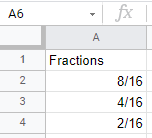# Display Fraction Without Reducing in Excel & Google Sheets

This tutorial demonstrates how to display a fraction without reducing it in Excel and Google Sheets.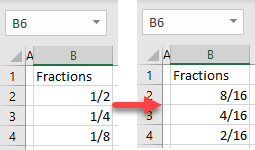## Display as Like Fractions

When you format a cell as a fraction, Excel automatically reduces it to the lowest possible denominator. For example, type 0 8/16 in cell B2.

For fractions between 0 and 1, start by typing a 0 so it’s recognized as a fraction. Otherwise, Excel recognizes is it as a date.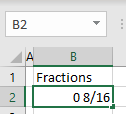As soon as you press ENTER on the keyboard, the value in cell B2 turns into 1/2. This happens because fractions are stored as decimals and displayed with the lowest possible denominator. In this case, the value behind the fraction is 0.5.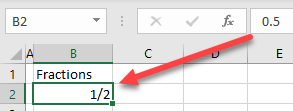Say you want to enter values 8/16, 4/16, and 8/16 in Column B. Excel automatically formats those fractions as 1/2, 1/4, and 1/8.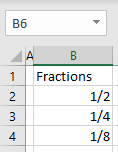To display the numbers as like fractions (with the same denominator) instead of reduced fractions, follow these steps:

1. Select (1) cells with fractions and in the Ribbon, (2) go to the Home tab, and (3) click on the format cells icon in the Number group.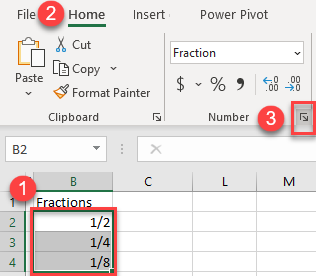1. In the Format Cells window, (1) select the Custom category, (2) enter # ??/16 in the Type box, and (3) click OK.
Note that this is the format for a two-digit denominator (16). If you want a three-digit denominator (like 100), enter #???/100, i.e., one question mark per digit.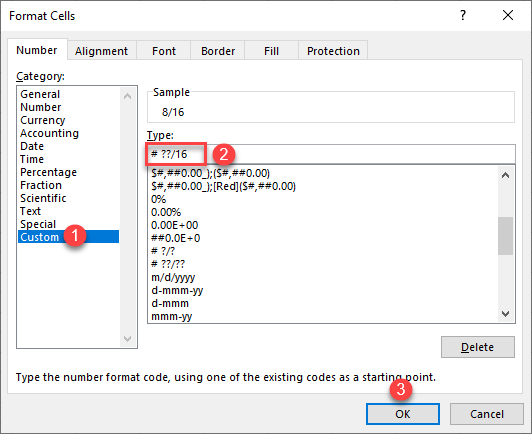As a result, all fractions use 16 as their denominator.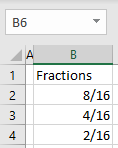## Display Fractions Without Reducing in Google Sheets

To display fractions without reducing in Google Sheets, you first have to enter all numbers as decimals, and then format them as fractions. Say you have the following decimal numbers and want to display them as fractions with denominator 16.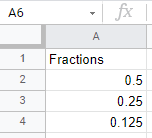1. Select the range of decimal numbers (A2:A4) and in the Menu, go to Format > Number > Custom number format.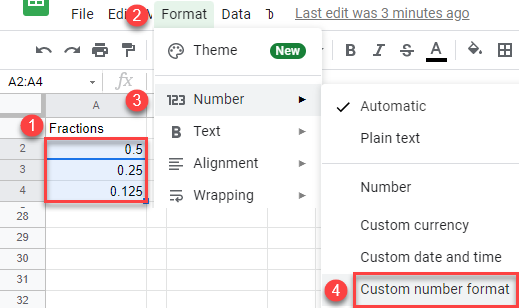1. In the format box, enter # ??/16 and click Apply.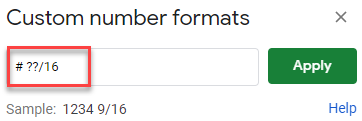As a result, all numbers are formatted as fractions without reducing.